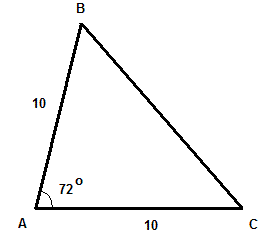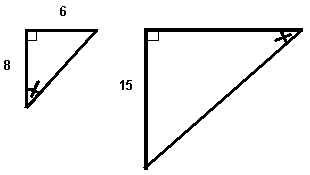# Grade 8 Problems and Questions on Triangles with Solutions and Explanations

Detailed solutions and full explanations to Grade 8 Problems and Questions on Triangles are presented.

 The lengths of two sides of a triangle are 20 mm and 13 mm. Which of these lengths cannot represent the length of the third side. 35 mm 10 cm 20 mm 45 mm Solution In any triangle, the sum of the lengths of two sides must be greater than the length of the third side. We are given two sides, the sum of their lengths is 20 + 13 = 33 mm Therefore the third side cannot be equal to 35 mm , 10 cm = 100 mm and 45 mm. ABC is an isosceles triangle. Find the size of angle ABC.. Solution The sum of the three angles in triangle ABC is equal to 180�. Hence 72 + angle ACB + angle ABC = 180 In the given isosceles triangle, angles ACB and ABC have equal measure. Hence 72 + 2 angle ABC = 180 2 angle ABC = 180 - 72 = 108 angle ABC = 54� The perimeter of an equilateral triangle is equal to 210 cm. What is the length of one side of this triangle? Solution The three sides of an equilateral triangle have equal lengths. If x is the length of one side of an equilateral triangle, then its perimeter is equal to 3x. Hence 3x = 210 x = 70 cm Find x so that the triangle shown below is a right triangle.. Solution Use Pythagora's theorem. (12x)2 + (16x)2 = 102 144 x2 + 256x2 = 100 400 x2 = 100 x2 = 1/4 x = 1/2 What will be the vertices of the triangle obtained by reflection on the a axis of the triangle defined by the vertices (1,2), (2,-3) and (4,-1)? Solution When a point of coordinates (x , y) is reflected on the x axis, its y coordinates changes sign and the coordinates become (x , - y). Hence when the vertices of the given triangle are reflected on the x axis, they become. (1,-(2)), (2,-(-3)) and (4,-(-1)) Simplify (1,-2), (2,3) and (4,1) The two triangles shown below are similar. Find the length of the hypotenuse of the larger triangle.. Solution In similar triangles, the corresponding sides have lengths in the same proportion. If h is the hypotenuse of the small traingle and H is the hypotanuse of the larger traingel, then 8 / 15 = h / H We now use Pythagora's theorem to find h. h2 = 82 + 62 = 100 Solve for h: h = 10 Substitute h in the above equation to find H 8 / 15 = 10 / H Cross multiply 8H = 150 H = 18.75 A 13 foot ladder is leaning against a vertical wall. The lowest point of the ladder is 4 feet from the wall. What is the height of the point where the ladder touches the wall? (round your answer to the nearest tenth of a foot). Solution The ladder, vertical wall and the ground make a right triangle whose hypotenuse is the ladder with length equal to 13 feet. One side of this triangle is 4 feet. If x is the other side, Pythagora's theorem may be used to find its length as follows x2 + 42 = 132 Solve for x x2 = 132 - 42 = 153 x = 12.4 feet (rounded to the nearet tenth) x is also the height of the point where the ladder touches the wall. The length of the hypotenuse of a right triangle is 40 cm. The size of one of its angles is 45 degrees. What are the exact lengths of the other two sides of the triangle? Solution A right triangle with the size of one angle equal 45� will also have the second angle with size equal to 45� since the sum of all 3 angles must be equal to 180�. So this triangle is right and isosceles and therefore its side lengths are equal. Let x be the length of one of its side. Use Pythagora's theorem. x2 + x2 = 402 2 x2 = 1600 x2 = 800 = 2�400 x = sqrt(2�400) = 20 sqrt(2) Triangle ABC is an isosceles triangle. The length of the base is 20 meters and the corresponding height is 24 meters. Find the perimeter of ABC. (round your answer to the nearest tenth of a meter). Solution The isosceles triangle ABC is shown below. Height AM is drawn. Triangles AMB and AMC are congruent since they have two congruent sides AB and AC and AM is common. Plus angles B and C are equal in size and the right angles are also equal. Hence the lengths of AM and CM are equal and therefore the length of MC is equal to 10 meters.. We now use Pythagora's theorem to find length x of side AB x2 = 242 + 102 = 676 x = 26 meters. The perimeter of the triangle is equal to length of side AC + length of side AB + length of side BC = 26 + 26 + 20 = 72 meters A triangle has an area of 90 square cm. Find the length of the base if the corresponding base is 3 cm more then the height. Solution Let b be the length of the base and h be the length of the height. Hence the area A of the triangle is gievn by A = (1/2) � base � height = (1/2)� b � h = 90 length of base is 3cm more that the height. Hence b = h + 3 Substitute b by h + 3 in the equation (1/2)� b � h = 90 to write (1/2))� (h + 3) � h = 90 Cross multiply the above equation (h + 3) � h = 180 Expand and rewrite with right side equal to 0. h2 3h - 180 = 0 Factor and solve the equation. (h - 12)(h + 15) = 0 h = 12 b = 12 + 3 = 15 cm The perimeter of a triangle is 74 inches. The length of the first side is twice the length of the second side. The third side is 4 inches more than than the first side. Find the length of each side of the triangle. Solution Let x be the length of the second side. The first side is twice the second. Hence the first side is equal to 2x The third side is 4 inches more that the first side. Hence the third side is equal to 2x + 4 The third side is 4 inches more that the first side. Hence the third side is equal to Perimeter = side 1 + side 2 + side 3 = 2x + x + 2x + 4 = 5x + 4 But perimeter is known to be 74. Hence 5x + 4 = 74 Solve for x 5x = 70 Solve for x x = 14 Length of different sides side 1: 2x = 2 * 14 = 28 inches side 2: x = 14 inches side 3: 2x + 4 = 28 + 4 = 32 inches. Determine the area of the triangle enclosed by the lines y = -4, x = 1 and y = -2x + 8: Solution The three lines are drawn in a standard system of axes and the three vertices of the triangle are A, B and C. To find the area of the the triangle, we need to find the lengths of the height and base. To find the height and base , we need to find the coordinates of points A and C.. Point A is the intersection of lines x = 1 and y = -2x + 8. So point A has x coordinates x = 1. Hence the y coordinate is found by substitution x by 1 in the equation of line y = -2x + 8. y = -2(1) + 8 = 6 Let yA be the y coordinate of point A and yB the y coordinate of point B. The length of height AB is given by. AB = |yA - yB| = |6 -(-4)| = 10 Point B is the intersection of lines y = - 4 and y = -2x + 8. So point B has y coordinates y = - 4. Hence the x coordinate is found by substitution y by - 4 in the equation of line y = -2x + 8. - 4 = -2x + 8 Solve for x. x = 6 Let xB be the x coordinate of point B and xC the x coordinate of point C. The length of base BC is given by. BC = |xC - xB| = |6 - 1| = 5 The area A of the triangle ABC is given by. (1/2) � AB � BC = (1/2)� 10 � 5 = 25 square units Show that the the triangle with vertices A(-1,6), B(2,6), C(2,2) is a right triangle and find its area. Solution We first find the square of the distances between the points and then use the converse of Pythagora's theorem to see if the triangle is right AB2 = (2 - (-1))2 + (6 - 6)2 = 9 , hence AB = 3 BC2 = (2 - 2)2 + (2 - 6)2 = 16 , hence BC = 4 CA2 = (-1 - 2)2 + (6 - 2)2 = 25 , hence CA = 5 It is clear that CA2 = BC2 + AB2 which is Pythagora's theorem. So triangle ABC is a right triangle and its hypotenuse is CA (longest of all 3 sides). It area A is given by (1/2) � AB � BC = (1/2) � 3 � 4 = 6 square units